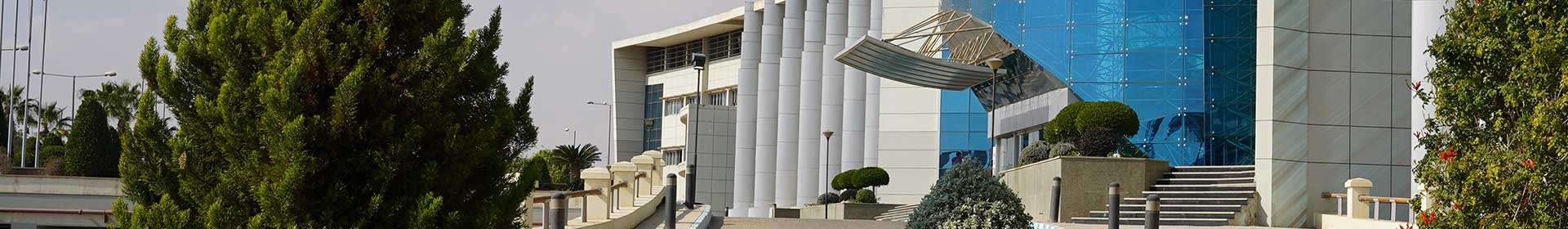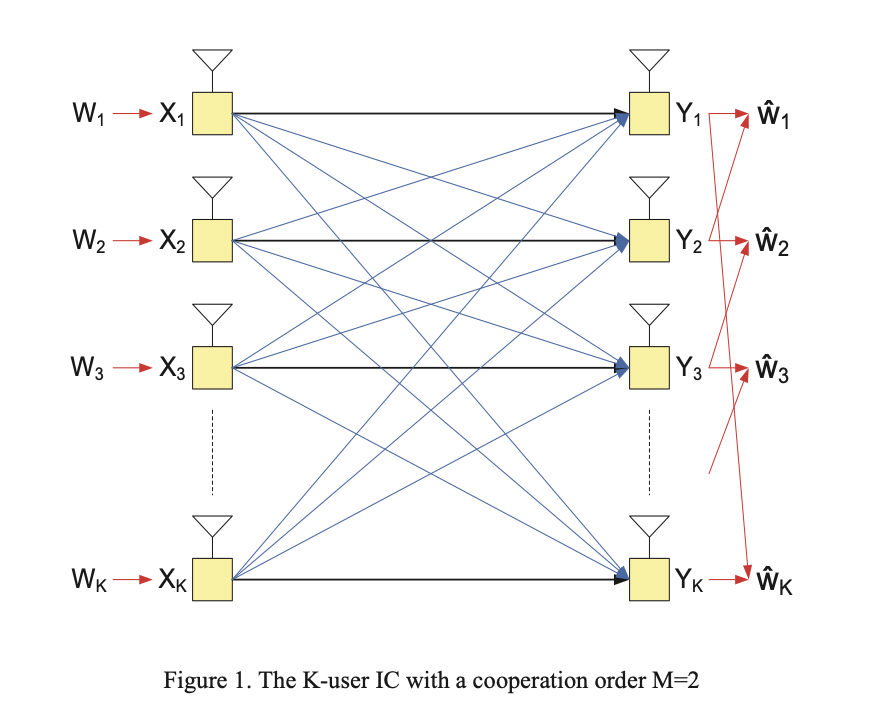Conference Paper

# Achievable degrees of freedom of the K-user interference channel with partial cooperation

By
Naguib A.A.
Elsayed K.
Nafie M.

In this paper, we consider the K-user interference channel with partial cooperation, where a strict subset of the K users cooperate. For the K-user interference channel with cooperating subsets of length M, the outer bound of the total degrees of freedom is KM/(M+1). In this paper, we propose a signal space-based interference alignment scheme that proves the achievability of these degrees of freedom for the case K= M+2 The proposed scheme consists of a design for the transmit precoding matrices and a processing algorithm which we call the Successive Interference Alignment (SIA) algorithm. The decoder of each message uses the SIA algorithm to process the signals received by the M cooperating receivers in order to get the maximum available degrees of freedom. © 2011 IEEE.